# Catch up on the latest AI articles# Augmentation To Retain Critical Information

3 main points
✔️ Avoids the lack of information that existed in state-of-the-art Augmentation
✔️ Proposes a simple, highly versatile method
✔️ Achieves accuracy on a wide variety of tasks while keeping computational costs low

written by Chengyue GongDilin WangMeng LiVikas ChandraQiang Liu
(Submitted on 23 Nov 2020)

Subjects: Computer Vision and Pattern Recognition (cs.CV)code：The images used in this article are either from the paper or created based on it.

## first of all

Data Augmentation (DA) is an essential technique for training current deep learning models, which is expected to avoid overfitting, model robustness, reduce model sensitivity to images, increase training data, improve model generalization, and avoid imbalance in samples. Many people are using DA for this purpose. However, DA inevitably introduces noise and ambiguity into the learning process. Look at the figure below. Random Cutout((a2) and (b2)) and RandAugment((a3) and (b3)) destroy the main characteristic information of the original image needed for classification and create an augmented image with wrong or ambiguous labels.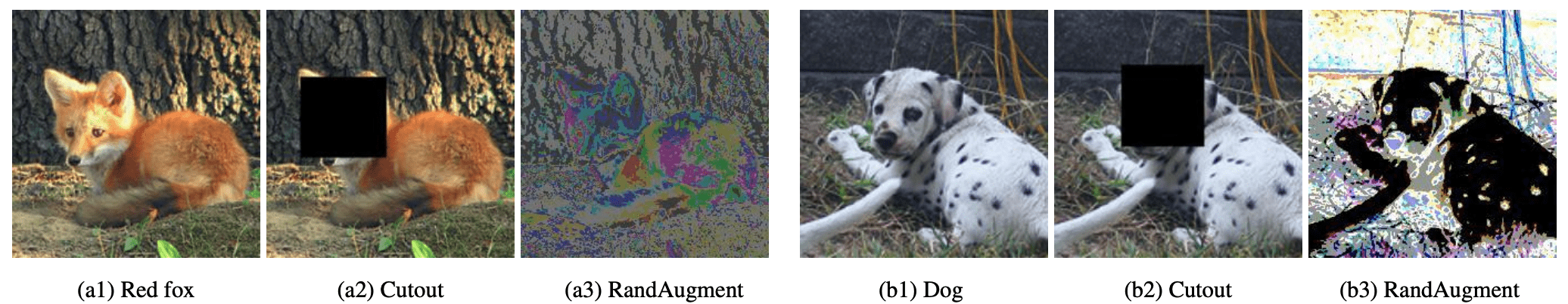The paper we present in this paper is an attempt to improve the DA It will increase the fidelity of DA images by a simple and effective method. The idea is to use a saliency map to detect important regions on the original image and then preserve these informative regions during DA. The idea is that this information preservation strategy can generate more faithful training examples. Essentially, I think it is in the same place as Attentive CutMix.

## Data expansion (since it includes a review)

We focus on label-invariant DA, where x is the input image and DA generates a new image $x'$=$A(x)$ with the same label as x. Here, A represents a label-invariant image transformation, which is usually a probability function. Let's take a quick look at the state-of-the-art DA, which is widely used in cutting-edge computer vision research, as well as a review.

cutout

The training data is created by randomly masking rectangular regions of the input image. A small square region is randomly selected in the image and the pixel value of this region is set to 0, but the label remains the same. Cutout prevents the model from relying on the visual features of a particular region and aims to make better use of the global information in the image.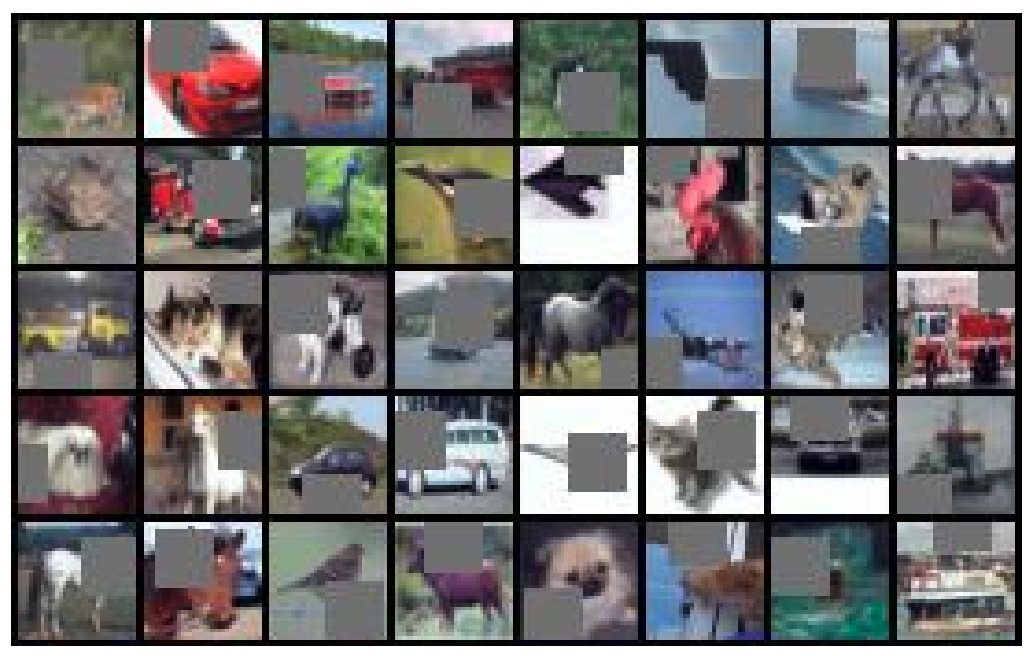Random Erasing

While Cutout uses fixed squares and the replacement values are all the same, Random Erasing has all random replacement values for the length and width of the mask area and the pixel values within the area.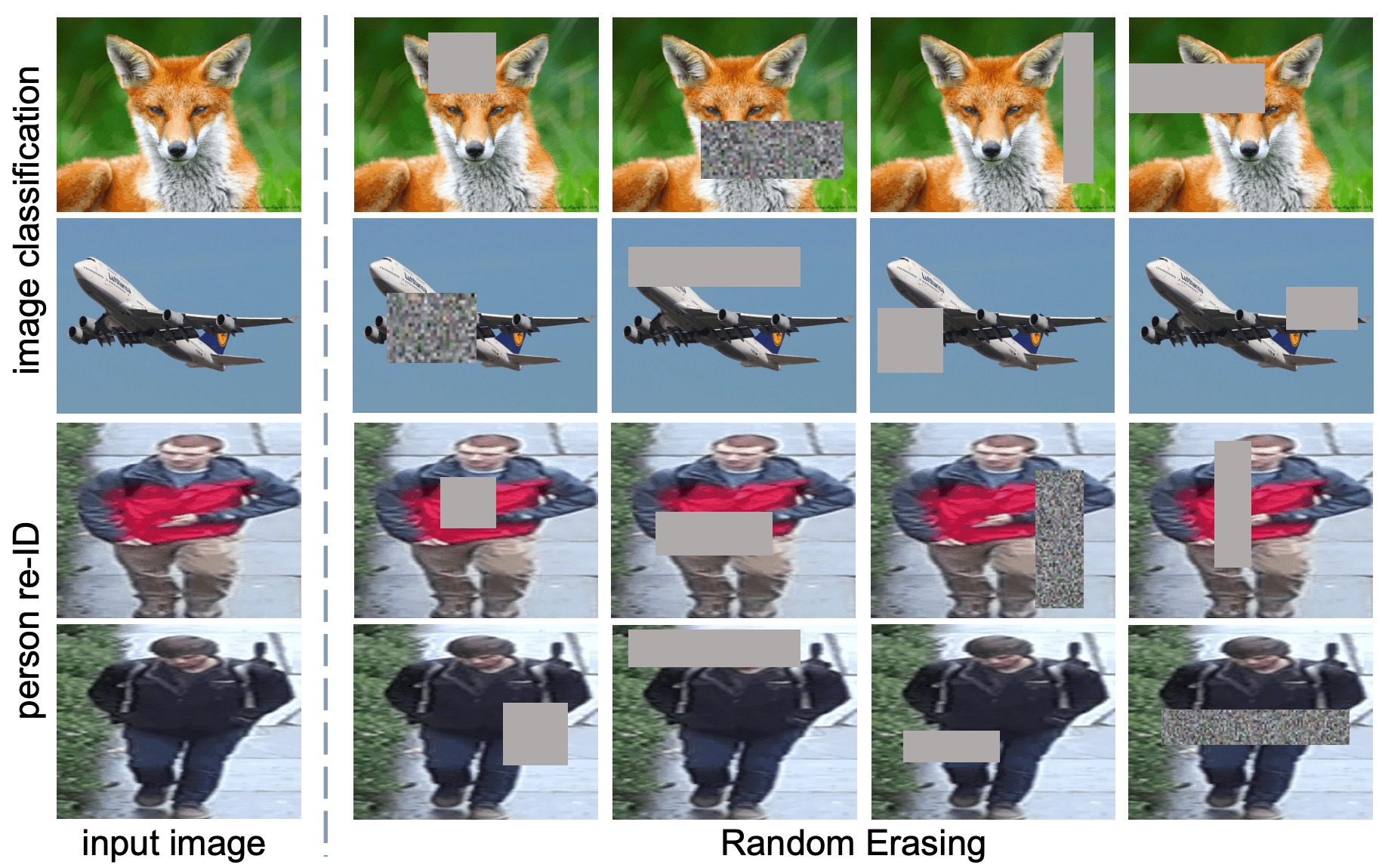Hide-and-Seek

The image is divided into a grid of S × S patches, each of which is masked with a certain probability (0.5). The hiding of the patches is changed for each epoch, and this allows us to learn multiple regions associated with an object.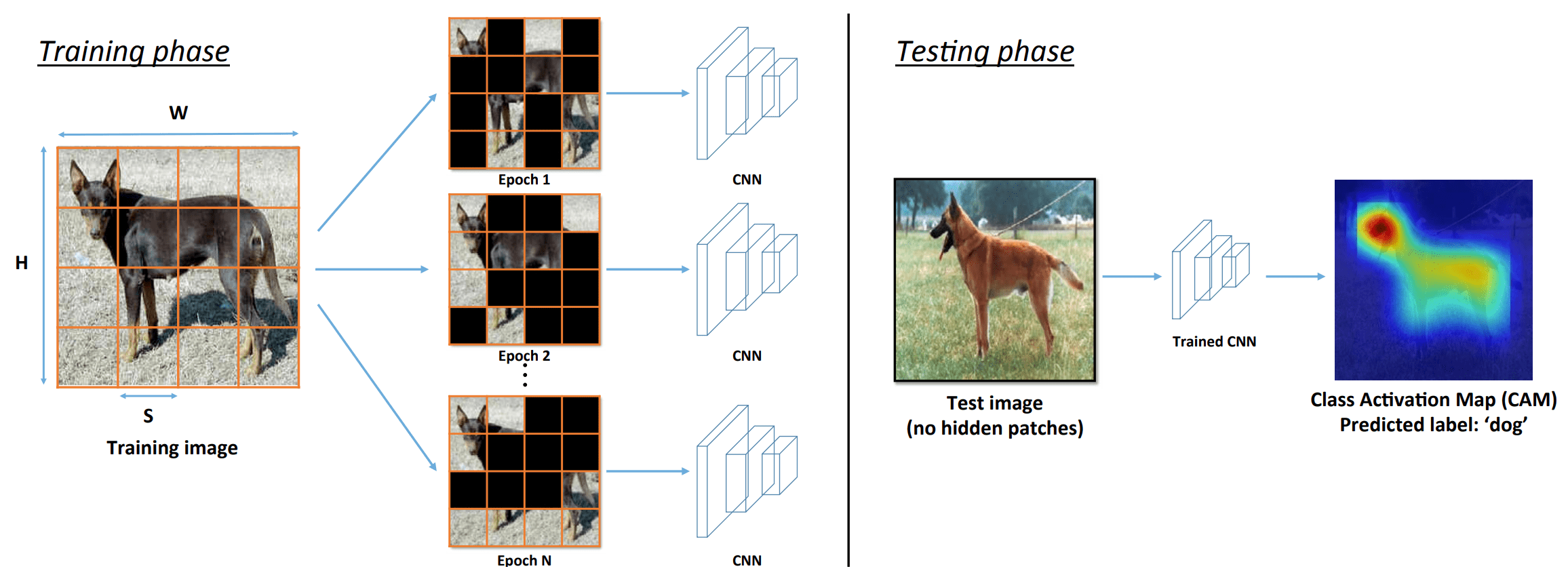Mixup

It fuses two randomly selected images from the dataset in a certain ratio, including the tag values. For example, we will fuse a dog and a car in about 0.50%.

So far, these methods have been proposed in 2017.

CutMix

This method combines Cutout, Random erasing, and Mixup with intermediate blending changes to select a small area for masking, but mask the other images so that they overlap that area.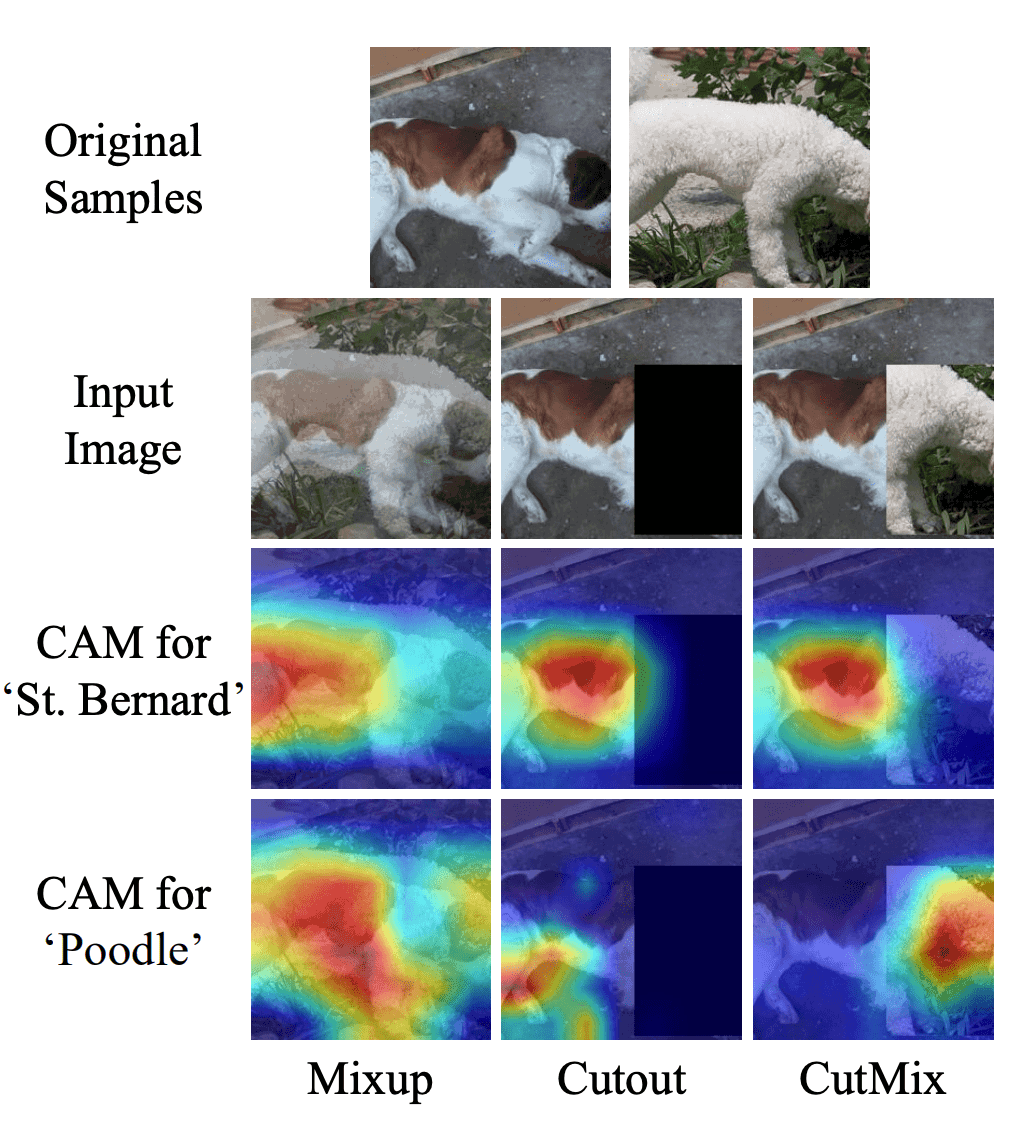The above method has a problem that important areas are completely masked because the masking area is selected randomly, but GridMask improves this problem. GridMask improves this problem by masking the area with squares arranged in a predetermined grid.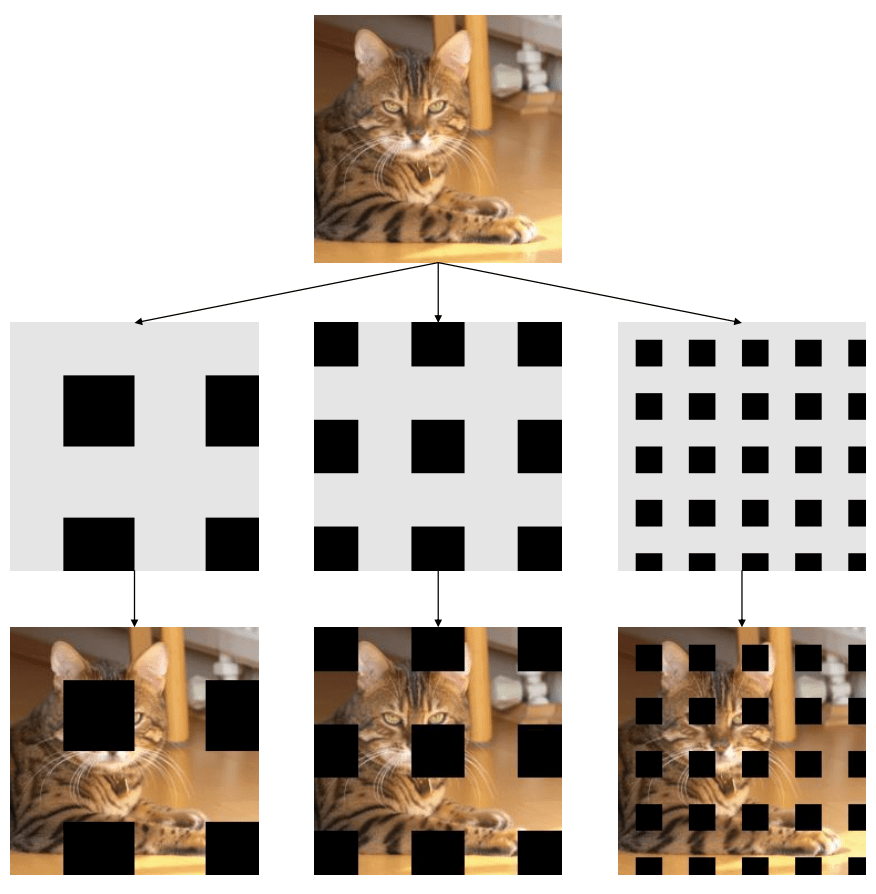It is a modified version of GridMask. Simply speaking, we propose FenceMask as a better shape for masking.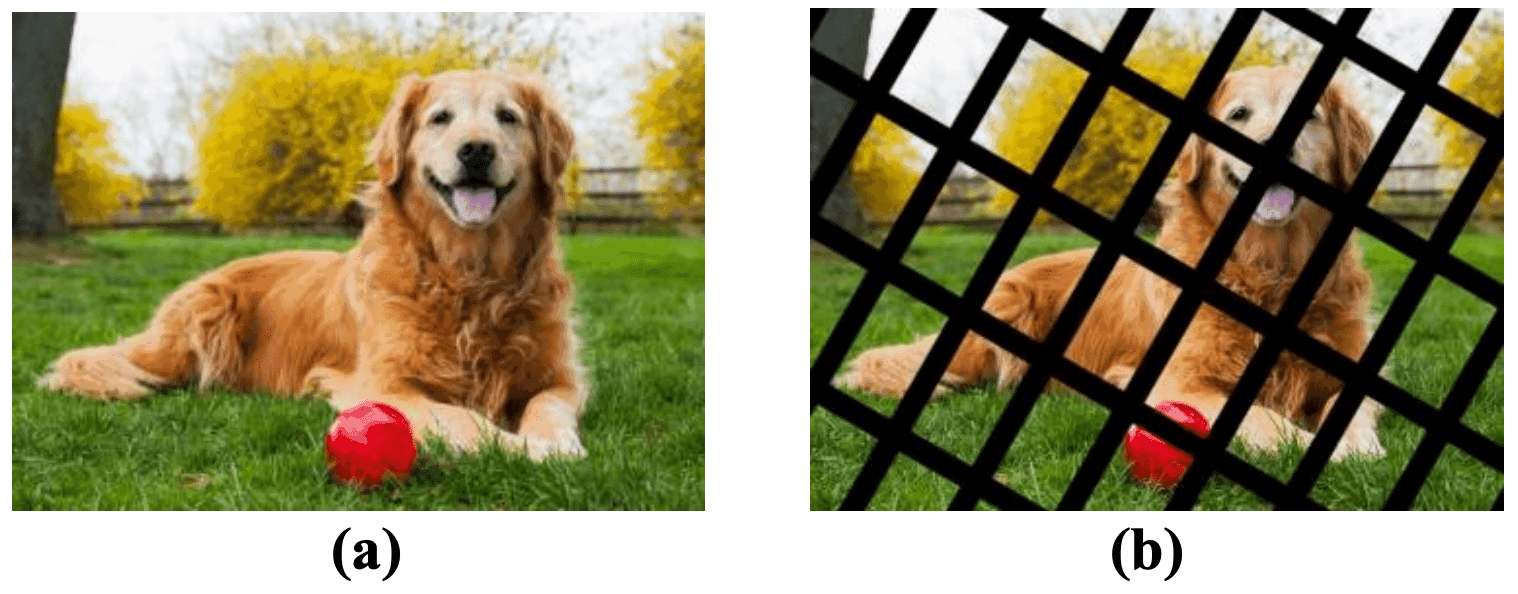AutoAugment(2019~)

A method for finding the best strategy for selecting and combining different label invariant transformations (rotation, color inversion, etc.) and learning a policy for selecting appropriate extension parameters; a method for finding the best combination using DA techniques.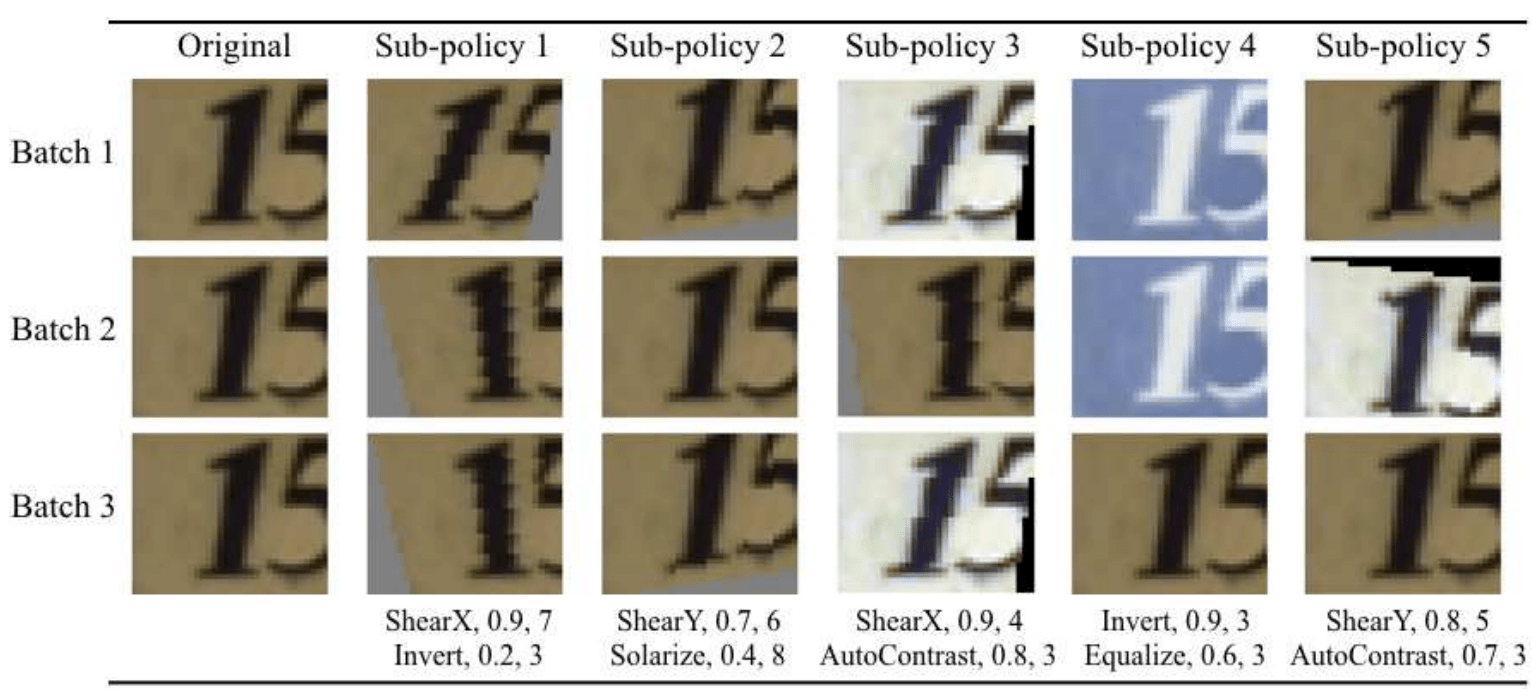Fast Autoaugment

This is a faster AutoAugment. We also proposed a method to avoid GPU consumption of learning many times at the same time, which is 100-1000 times faster.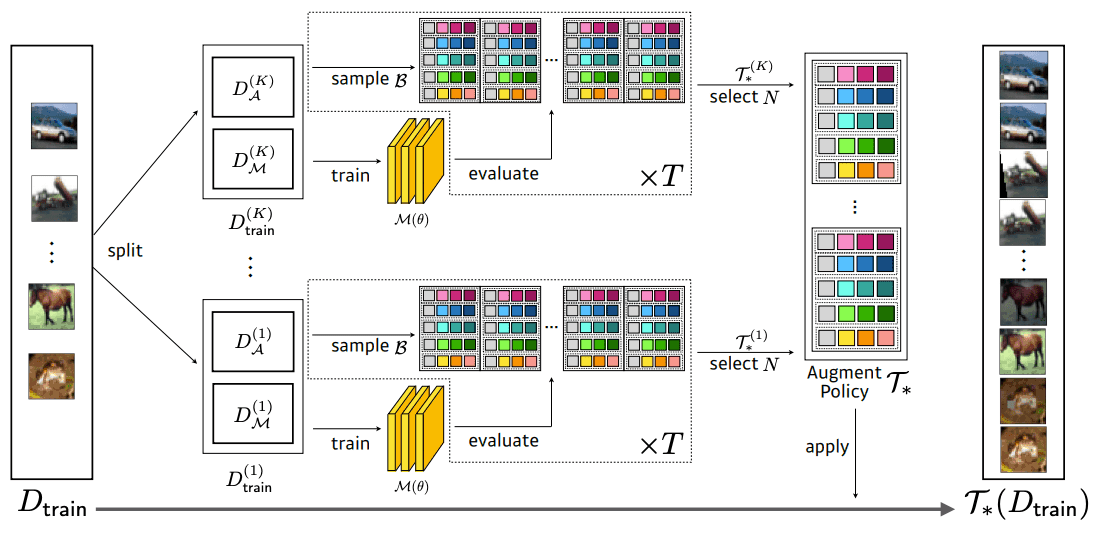RandAugment

RandAugmentation improves on the shortcomings of AutoAugment, such as increased training complexity and computational cost, and the inability to adjust the strength of regularization depending on the size of the model or dataset. RandAugmentation can be lumped in with the model training process.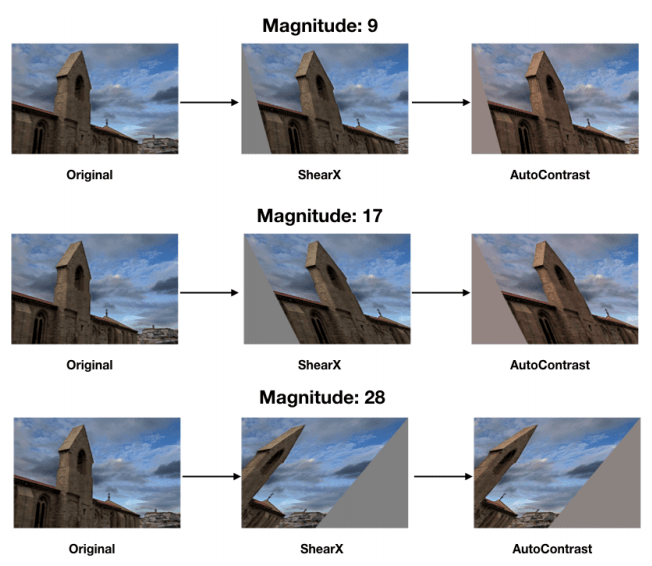This time's method is an evolution of these: the problem of "important areas being completely masked", which was also pointed out in Gridmask, and the lack of information that is not grasped by more and more automatically optimal DA due to the evolution of AutoAugment. The content of this paper is to make improvements to these problems. You can also read the authors' thoughts in the following discussion.

To investigate the above idea, the authors conduct experiments on the CIFAR-10 dataset using two methods, Cutout and RandAugmentation, and analyze the relationship between the strength and accuracy of the DA methods. The strength of Cutout is controlled by the length of Cutout, and the strength of RandAugmentation is controlled by the magnitude of Distortion. The experimental results are shown in the following figure.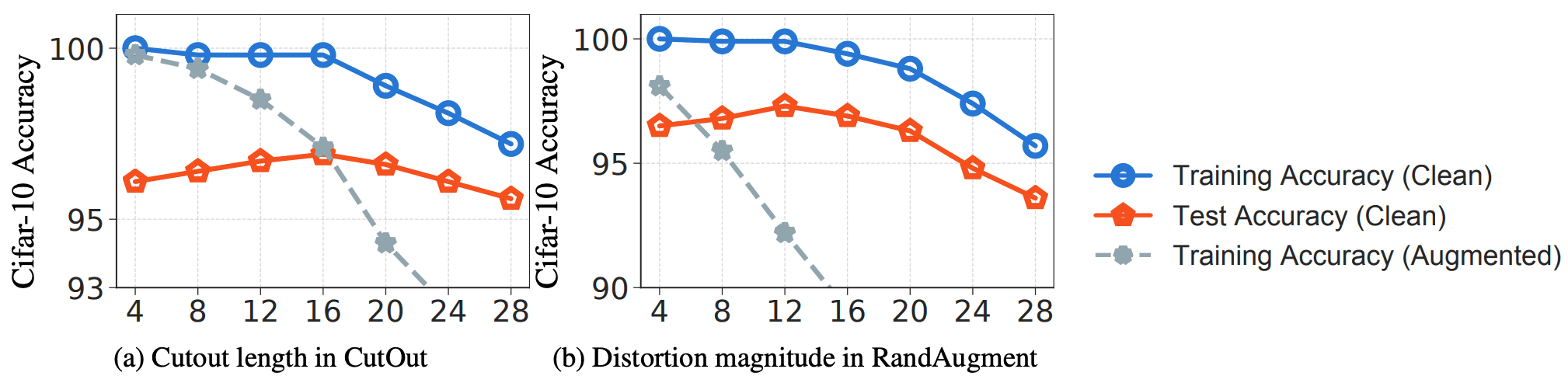As usually expected, in both cases the generalizability (the gap between the training and testing accuracy of the original data) increases with the size of the transformation. However, when the size of the transformation is too large (for Cutout ≥ 16 and RandAugmentation ≥ 12), the learning (blue line) and testing (red line) accuracies start to decrease. This indicates that the DA data is no longer a faithful representation of the pure training data and that the learning loss on the DA data does not form a good substitute for the learning loss on the pure data.

## proposed method

The idea of KeepAugment is to measure the importance in the image via saliency map so that the region with the highest importance score is retained after DA, as shown in a4 and b4. for Cutout, it is achieved by not cutting the important regions (a5, b5 ), and for image-level transformations such as RandAugmentation, we achieve this by pasting the important regions on top of the transformed image (a6, b6).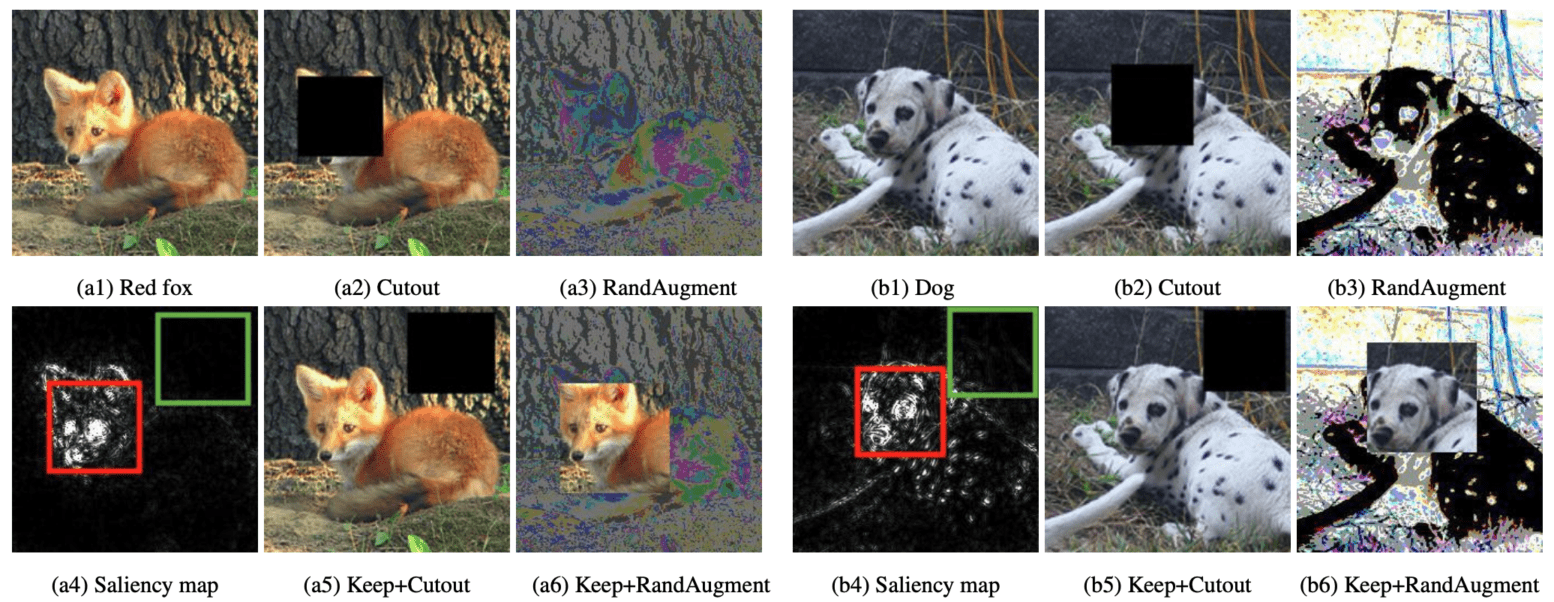From the above, KeepAugment can be divided into two steps: computing the saliency map and retaining the important rectangular regions. Retention of important rectangular regions is divided into "Selective-Cut" and "Selective-Paste" according to DA methods.

### Saliency map

KeepAugment uses a general gradient method to obtain the saliency map. Specifically, let $g_{ij}$(x, y) be the saliency map when a pixel (i, j) in image x is given a label y. Then, for a region S in the image, its importance score is defined as follows.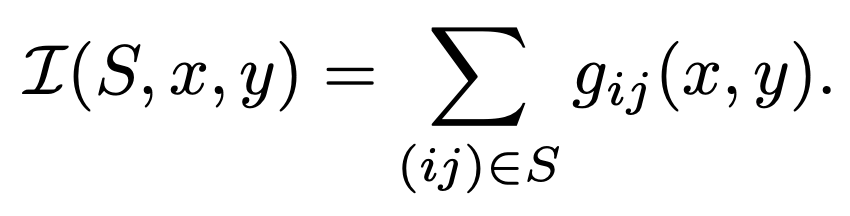Specifically, given an image x and its corresponding label logit value y(x), KeepAugment sets $g_{ij}$(x, y) to the absolute value of the gradient $|∇_(x)l_{y(x)}|$. For RBG images, we use the maximum value of the channel to find one significant value for each pixel (i, j).

### Selective-Cut

KeepAugment controls the DA fidelity in the DA method by ensuring that the importance score of the region to be deleted is not too large. This is actually achieved by Algorithm 1(a) in the figure below. In Algorithm 1, the region S to be cut is randomly sampled until its importance score I(S,x,y) is smaller than a given threshold τ.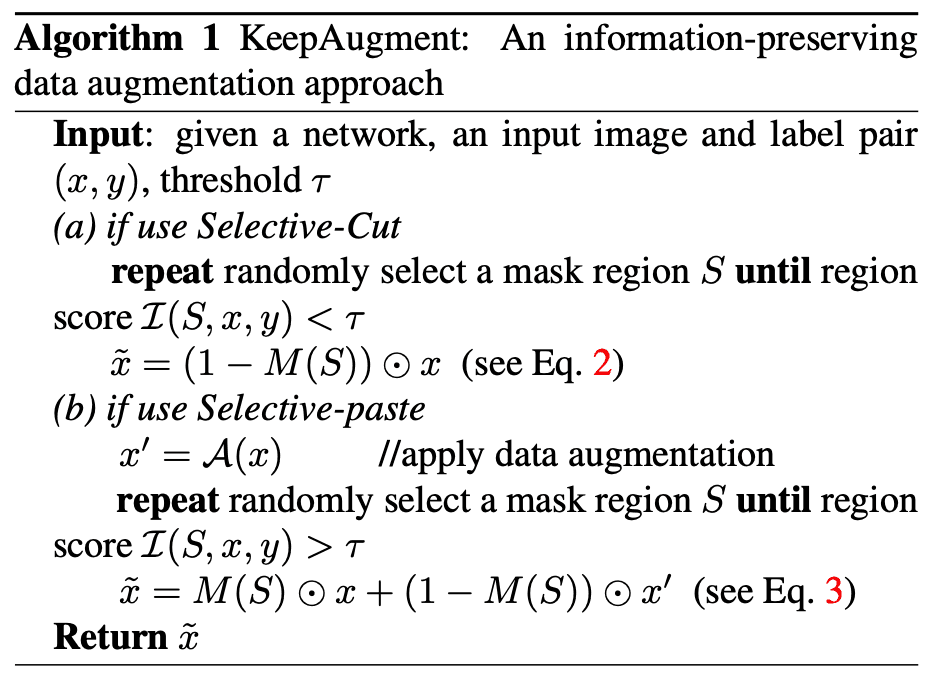where $M(S)$ = $[M_(ij)(S)]_(ij)$ is the binary mask of S and $M_(ij)$= $I((i, j) ∈ S)$.

### Selective-Paste

Since the image-level transformation modifies the entire image together, we ensure the fidelity of the transformation by attaching random regions with high importance scores. Algorithm 1(b) shows how this is achieved in practice, by plotting the extended image-level data $x_0$= A(x), uniformly sampling the region S satisfying I(S, x, y) > τ for a threshold τ, and pasting the region S of the original image x into $x_(0)$. we achieve the following.Similarly, $M_(ij)$= $I((i, j) ∈ S)$ is the binary mask of region S.

## Efficient implementation of KeepAugment

KeepAugment needs to compute the saliency map at each training step by backpropagation. Computing it directly would double the computational cost. Here, the authors propose an efficient method to compute the saliency map.

Based on the low-resolution approximation, the authors perform the following operations

1. For a given image x, we first generate a low-resolution copy and compute its saliency map.
2. Map the low-resolution saliency map to the corresponding original resolution.
This allows, for example, ImageNet to achieve a threefold reduction in computational cost by reducing the resolution from 224 to 112, which greatly speeds up the computation of the saliency map.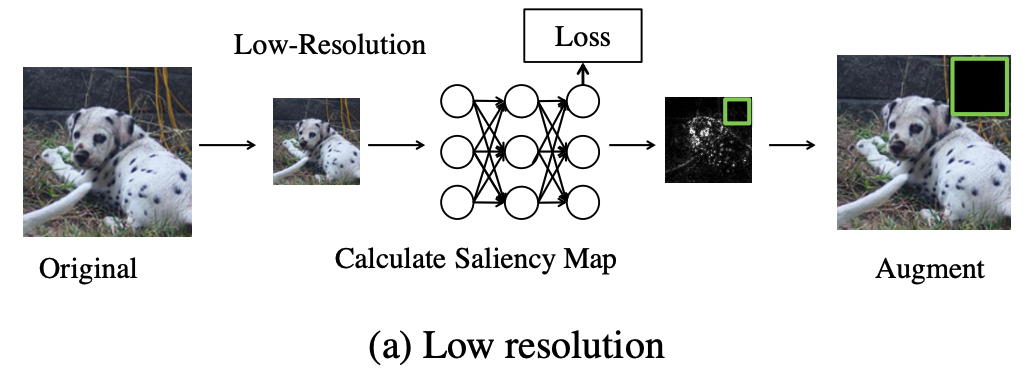Another idea, based on the initial loss approximation, is to add a loss to the initial layer of the network and use this loss to create a saliency map. In effect, an additional averaging pooling layer and the linear head are added after the first blocks of the network have been evaluated. The learning objective is the same as for the Inception Network. The neural network is trained with standard and auxiliary losses. This idea allows us to reduce the computational cost by a factor of 3 when computing the saliency map.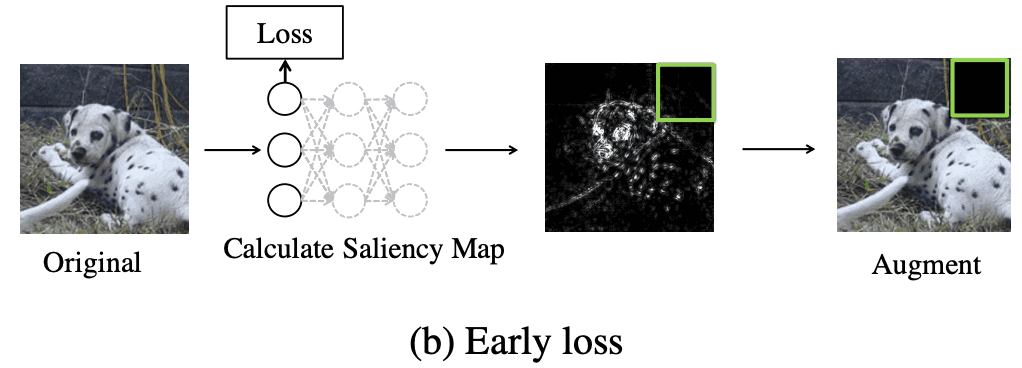Throughout the actual experiments, neither approximation strategy caused any degradation in performance.

## Experiment

The authors will experimentally show that KeepAugment achieves improved accuracy in a variety of deep learning tasks, including image classification, semi-supervised image classification, multi-view and multi-camera tracking, and target detection. We will pick a few of them and take a look at them.

### Dataset

The following four data sets were used.

• CIFAR-10
• ImageNet
• COCO 2017
• Market1501

・CIFAR-10 Classification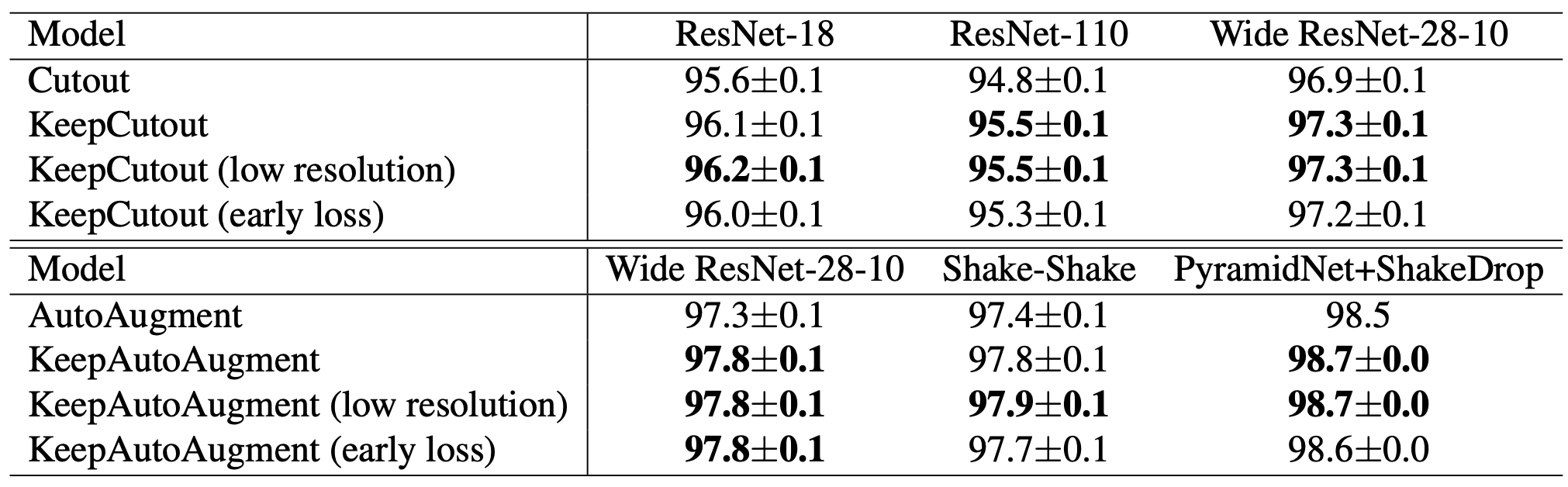You can see that accuracy improvement can be done by classification. There may have been more or less noise to the image. (It is calculated by the average value of multiple trials.)

・Training Cost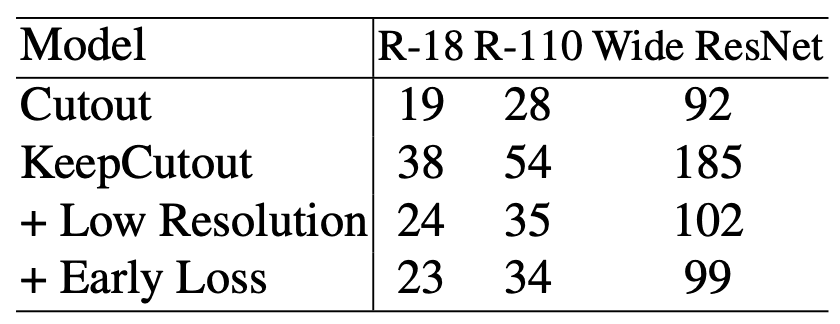In the classification, the Keep method contributed to the accuracy improvement, but when we look at the learning cost, a simple KeepCutout is almost twice as expensive as a regular Cutout. However, as we have already seen in the methodology, two approaches to reduce the learning cost have a strong effect on this. By using low resolution images and early loss to compute the saliency map, we can see that the accuracy is improved with a small increase in learning cost compared to Cutout.

Semi-Supervised Learning Semi-Supervised Learning

Here are the results: the expression 4,000 means that 4,000 images are labeled and the remaining 4,6000 images are unlabeled.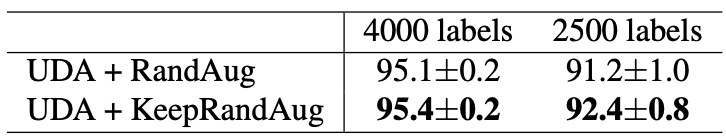We can see that the accuracy is consistently higher than RandAug. However, the improvement was slight.

Multi-View Multi-Camera Tracking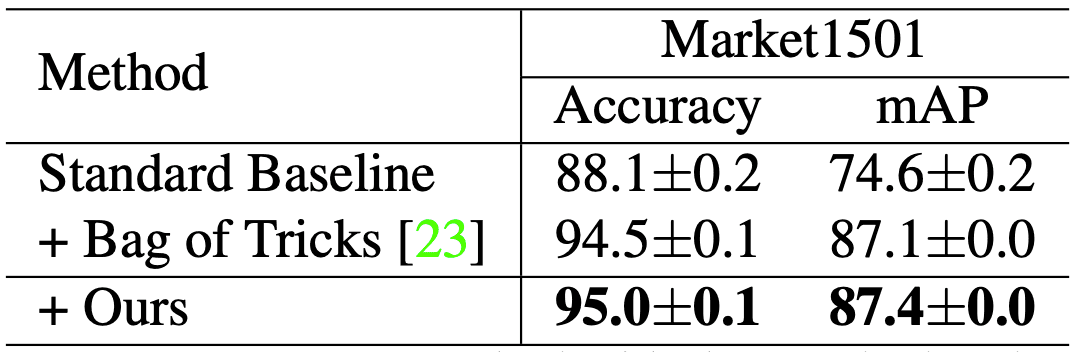The comparison between the baseline and other methods is given. It can be seen that the proposed method achieves the best performance.

Transfer Learning: Object Detection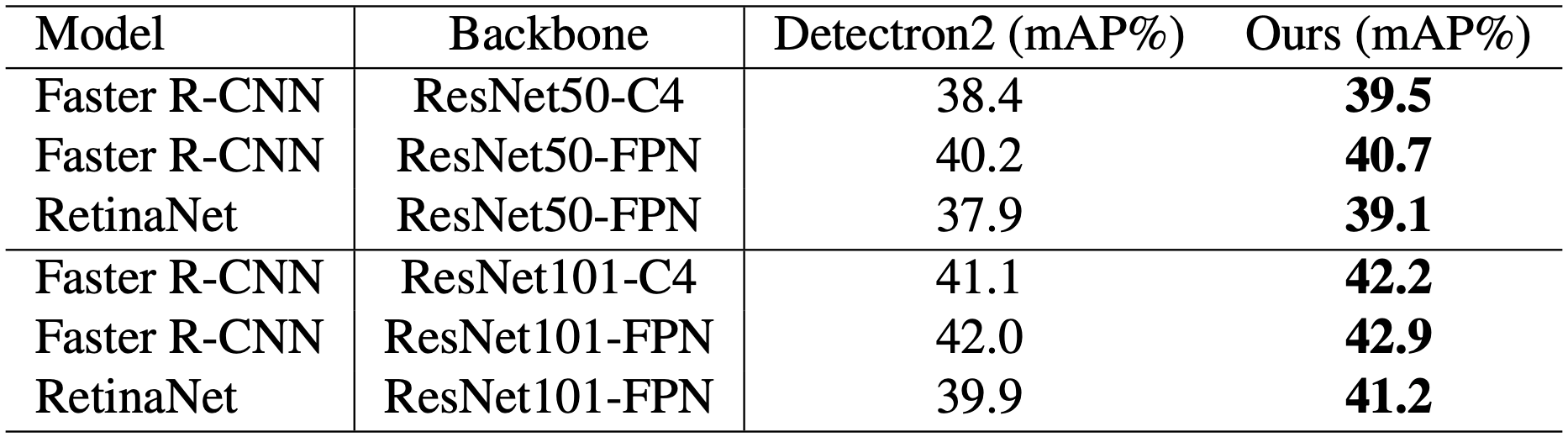We can see a consistent improvement over the baseline. Simply replacing the backbone network with the one trained by the proposed method improves the performance on the object detection task in COCO 2017 without any additional cost. In particular, the single-stage detector RetinaNet improves from 37.9 mAP to 39.1 and 39.9 mAP to 41.2 for ResNet-50 and ResNet-101, respectively.

However, ResNet50 + CutMix performed extremely poorly, with 23.7, 27.1, and 25.4 (mAP) for the C4, FPN, and RetinaNet settings, respectively. CutMix is a very effective DA method, but it may cause strong accuracy loss for some tasks and models Although CutMix is a very effective DA method, it may cause strong accuracy loss for some tasks and models.

## development

It can be said that empirical studies of the impact of DA fidelity and diversity have revealed that a good DA strategy needs to jointly optimize these two aspects. More recently, a number of other studies have also shown the importance of balancing fidelity and diversity. For example, " showed that performing the worst optimization or choosing the most difficult reinforcement strategy is effective, which demonstrates the importance of diversity.

To reduce the noise in CutMix, we consider extracting the most informative regions. The idea is the same as our method. However, the disadvantage of their proposed method, Attentive CutMix, is that it requires an additional pre-trained classification model as a teacher. Moreover, the advantage of our method is that it can be applied to more DA methods and tasks, which Attentive CutMix cannot do.

## summary

It has been suggested that existing DA methods are limited in their ability to improve overall performance due to the possibility of introducing noise and ambiguous samples. Therefore, the authors proposed to measure the importance of each region using a saliency map and avoid region-level DA methods that cut important regions (cutout) or perform image-level DA by pasting important regions from the original data (RandAugment).

It is quite intuitive and can be developed in the future. For example, we focus on label-invariant DA, but it seems that research could be done to apply it to label-variable as well. Not only that, but we can think of several ways to measure importance. We are now in the phase of how to perform correct DA and improve accuracy.

If you have any suggestions for improvement of the content of the article,